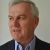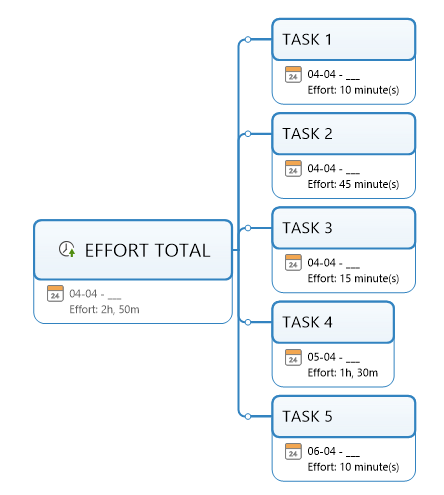# how to sum hours with MIndmanager

Darren shared this question ago

how to sum hours with Mindmanager with formule.

The propreties is an hours.

## Replies (1)2

I am not quite sure that I understand you correct, but...

This can be done via Advanced ribbon Autocalc.1

the duration is that format HH:MM:SS how to sum it .

09h30 min + 0h34 min + 4h45min on Microsoft Excel We can do it easly.1

Correct me if I’m wrong, but it looks like you have created a time topic property, or possibly a text one, in which you have entered the duration as hours and minutes.

Unfortunately MindManager’s ability to use formulas is limited to numeric topic properties, which don’t include time or date topic properties, or numbers in text properties. Put simply, MindManager can’t add a column of times expressed as hours and minutes in the same way Excel can.

You have three options:

• Enter the durations as minutes in a numeric topic property, and then sum these on the central topic using a formula.
• Enter the durations in a numeric topic property using whole numbers to represent hours and minutes, so 2 hours 30 minutes becomes 2.5. These numbers can also be summed on the central topic.
• Use the duration field in the task attributes. It appears that you have zero duration in terms of the task because you have set only a start date, and neither a due date or duration. You can set a duration in minutes (assuming all the durations are under 8 hours this would mean that the due date would be the set automatically to be the same as your start date). You could then roll up the durations to the central topic rather than use a formula.2

To add to my previous response, if you use the first option you could add a simple formula to divide the total number of minutes by 60, which would give you the total hours and the minutes as a decimal fraction of hours (which is how the second option will display).

I have also realised that you can end up with errors if you use the third option, because when MindManager rolls up the duration of subtopic tasks with durations of less than a day spread across multiple days it tends to round the result up to whole days. Instead of rolling up you can use a formula on the central topic to total the duration minutes of the subtopic tasks then divide by 60 to give you the total in hours and minutes as a fraction of hours.1

Thank you @alex for all.1

Thanks Darren. I should also state that in relation to the third option, MindManager's rollup Duration total is based on the duration from the beginning of the first task to the end of the last task, regardless of whether they overlap or whether there are gaps between them (see discussion in the following thread: https://community.mindmanager.com/topic/1772-inaccuracies-in-rolling-up-task-durations-of-less-than-a-day).

That's why using a formula on the central topic to sum the total durations of subtasks is a better alternative. An even better option would be to use Effort instead of Duration. This will roll up and sum the total Effort of the subtasks regardless of duration, gaps and overlaps. You would have to untick the link between Duration and Effort on each task and enter the latter manually, but like your original example the result would be shown in hours and minutes for total times over one hour. You also don't need to use a formula to sum the times, or to divide the total by 60:---